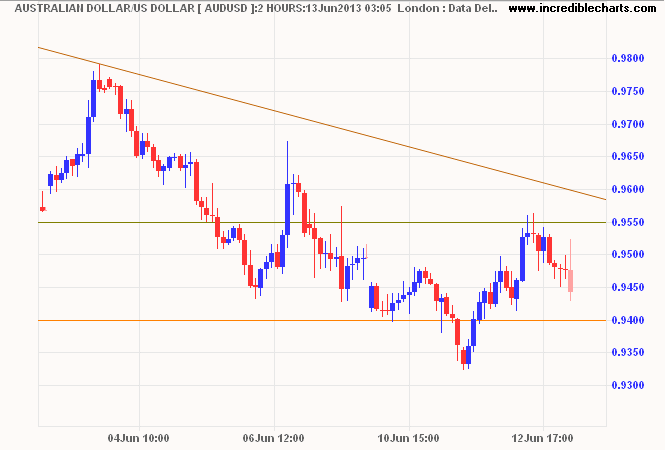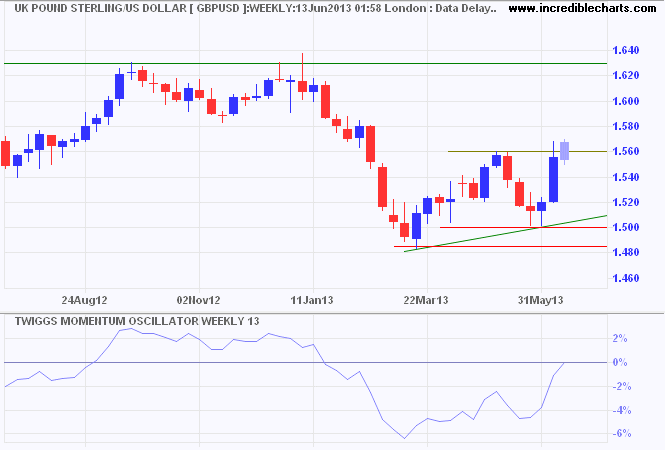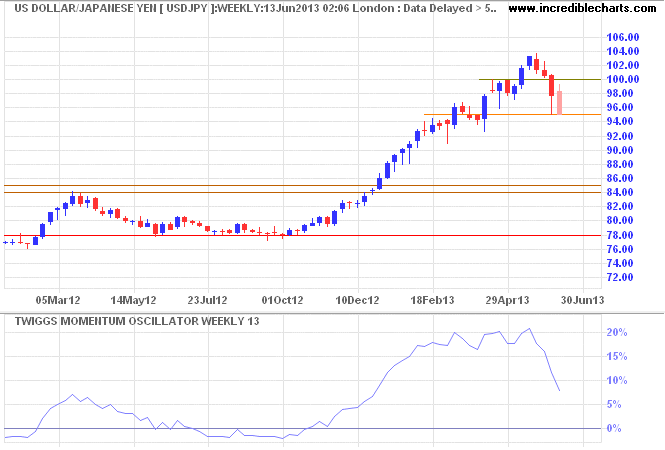# Forex: Aussie resistance, Yen falls

The Aussie Dollar rallied to \$0.955 on the 2-hour chart before encountering selling pressure. Expect a test of the 2011 low at \$0.94. Breach would indicate another decline. The next target is \$0.90*, with a long-term target of \$0.80*. Breakout above \$0.955 is unlikely, but would re-test resistance at \$0.98.* Target calculations: 0.94 – ( 0.98 – 0.94 ) = 0.90 and 0.95 – ( 1.10 – 0.95 ) = 0.80

Canada’s Loonie, however, respected support at \$0.96, heading for another test of resistance at \$0.99 or parity. 13-Week Twiggs Momentum below zero suggests continuation of the down-trend. Respect of resistance would indicate another decline, with a target of \$0.94*.* Target calculation: 0.97 – ( 1.00 – 0.97 ) = 0.94

The euro broke resistance at \$1.32 and is headed for \$1.37*. Breakout is some way off, but would offer a target of \$1.47*.* Target calculation: 1.37 + ( 1.37 – 1.27 ) = 1.47

Pound Sterling broke resistance at \$1.56, signaling an advance to \$1.63*. Recovery of 13-week Twiggs Momentum above zero would strengthen the bull signal.* Target calculation: 1.56 + ( 1.56 – 1.50 ) = 1.62

The greenback continues a strong correction against the Yen, but this is a secondary movement and the primary up-trend is unaltered. A 13-week Twiggs Momentum trough above zero would strengthen the signal. Recovery above resistance at ¥100 would signal a fresh advance with a target of ¥113*. Long-term target for the advance is the 2007 high at ¥125*.* Target calculations: (a) 104 + ( 104 – 95 ) = 113; (b) 100 + ( 100 – 75 ) = 125